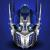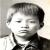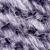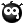## 二叉树的非递归遍历出问题

linbin0182 发布于 2013/04/15 13:23

@cut 你好，想跟你请教个问题：#include<stdio.h>
#include<stdlib.h>
typedef struct BTree{
int data;
struct BTree *lchild,*rchild;
}BiTNode,*BiTree;
typedef struct {
BiTree top,base;
int length;
}stack;//栈的结构定义
void CreatBinaryTree(BiTree &T)
{//构造一个二叉树（递归定义）
int ch;//以0表示空树
printf("输入结点：");
scanf("%d",&ch);
if(ch==0) T=NULL;//约定值为零的结点为空
else
{
T=(BiTree)malloc(sizeof(BiTNode));
if(!T) exit(1);
T->data=ch;
CreatBinaryTree(T->lchild);//递归
CreatBinaryTree(T->rchild);
}
}
void VistTree(BiTree &T)
{//输出结点
if(T!=NULL)
printf("%d ",T->data);
}
void InitStack(stack &s)
{//初始化一个栈
s.base=(BiTree)malloc((4)*sizeof(BiTNode));
if(!s.base)
exit(1);
s.top=s.base;
s.length=0;
printf("InitStack Successfully!\n");
}
int StackEmpty(stack &s)
{//判断栈是否为空
if(s.length==0||s.base==NULL)
return 0;
else
return  1;
}
void PushStack(stack &s,BiTree &p)//注意参数类型
{//入栈操作
//栈中依次存放的是二叉树中各结点，而且不打乱各结点之间的连接关系
if(s.length>=4)
s.base=(BiTree)realloc(s.base,(4+4)*sizeof(BiTNode));
if(!s.base)
exit(1);
s.top=p;
s.top++;
s.length++;
}
void PopStack(stack &s,BiTree &p)//注意参数类型
{//出栈操作
if(StackEmpty(s))
{
s.top--;
p=s.top;
s.length--;
}
}
void MiddleTree(BiTree &T,stack &S)
{//中序遍历二叉树非递归实现
BiTree p;
InitStack(S);p=T;
while(p!=NULL||StackEmpty(S))
{
if(p!=NULL)
{
PushStack(S,p);
p=p->lchild;
}
else
{
PopStack(S,p);
VistTree(p);
p=p->rchild;
}
}
}
int main(void)
{
BiTree T;
stack S;
CreatBinaryTree(T);
MiddleTree(T,S);
return 0;
}为什么输入数字1Enter 2Enter 3Enter 0Enter 0Enter 0Enter 0Enter得出的是31然后显示错误啊

10void InitStack(stack &s) ，你是c语言还是c＋＋,c++就用c++的代码书写方式，c就用c的。还说不乱？语言就乱用了。。。
0这个不是要自己调才有乐趣的么。

0https://github.com/burningsun/pecker_framework.git 这个是我蛋疼的时候写的，在data文件夹里的都是一些数据结构，自己看二叉树的遍历实现，有AVL树的实现和迭代器的遍历实现，三叉字符搜索树的实现和迭代器遍历实现，代码比较乱，怎么用的看vs工程里面testcode的代码，发现什么BUG也可以给我说